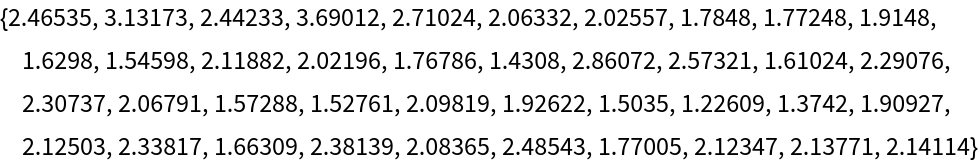#Function Repository Resource:

# ExtendedLatticeReduce

Compute a reduced basis for a set of vectors, along with a unimodular matrix that converts from the vectors to the reduced basis

Contributed by: Daniel Lichtblau
 ResourceFunction["ExtendedLatticeReduce"][lat] computes a reduced basis ℬ for the lattice of integer vectors lat, returning the pair {𝒰,ℬ}, where 𝒰 is a unimodular conversion matrix satisfying 𝒰.lat=ℬ.

## Details and Options

The input lattice lat must be a matrix of integers and/or rational numbers. Gaussian integers and rationals are also permitted.
The conversion matrix is square and unimodular (it has determinant equal to ±1).
ResourceFunction["ExtendedLatticeReduce"][lat,"ReductionLambda"λ] will use the value λ for the reduction ratio in the LLL algorithm.
The reduction ratio λ must be in the range 1/4 ≤ λ<1.
The value of the "ReductionLambda" option setting can affect the speed and quality of the reduction. A lower value will typically give a faster computation, but result in a lattice that is further from being fully reduced (it will of course be reduced in the LLL sense appropriate for the parameter setting).

## Examples

### Basic Examples (5)

Create an integer lattice comprised of two columns of large integers augmented on the right by an identity matrix:

 In:=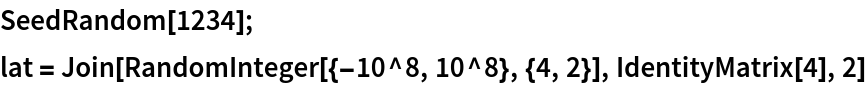Out=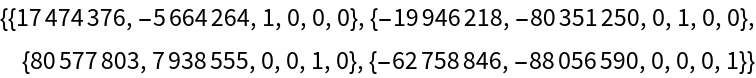Reduce the lattice:

 In:=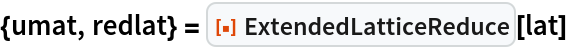Out=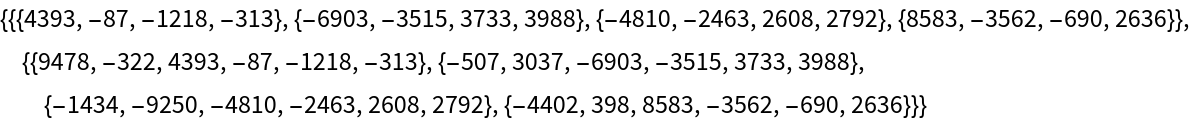Check the conversion matrix equation:

 In:=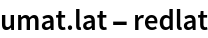Out=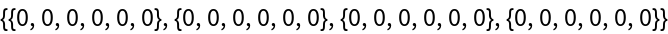Check that the conversion matrix is unimodular:

 In:=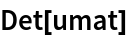Out=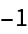Check that LatticeReduce gives the same reduced lattice (this is not strictly necessary):

 In:=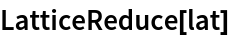Out=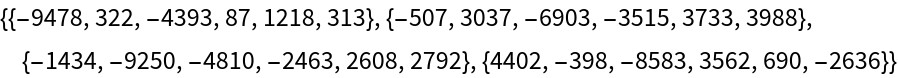### Scope (2)

Create a rational lattice:

 In:=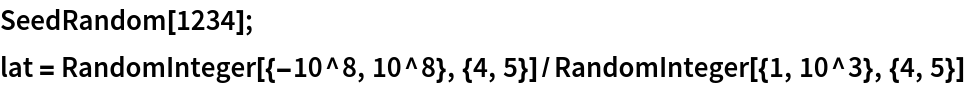Out=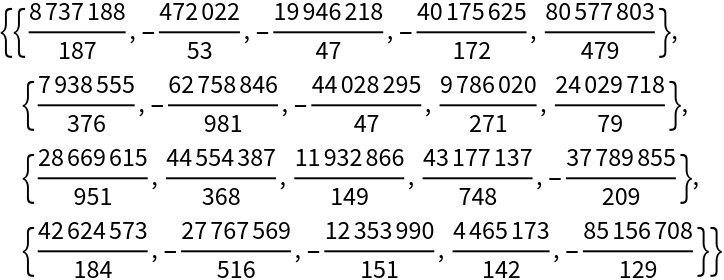Compute a reduction of the lattice along with the corresponding unimodular conversion matrix::

 In:=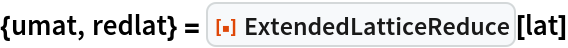Out=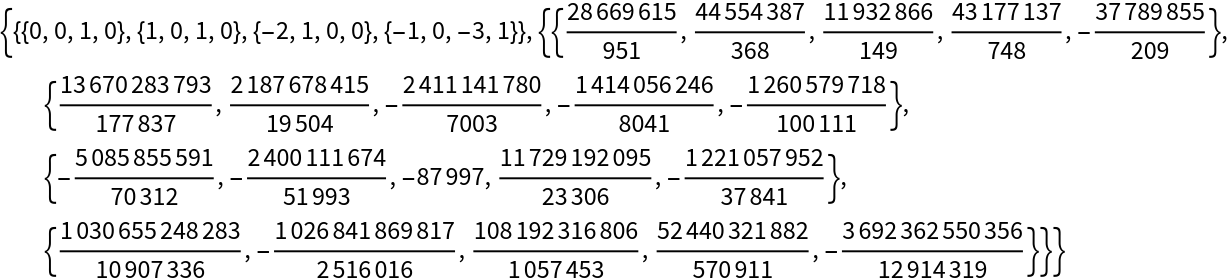Reduce a lattice of Gaussian integers with larger entries in the leftmost two columns:

 In:=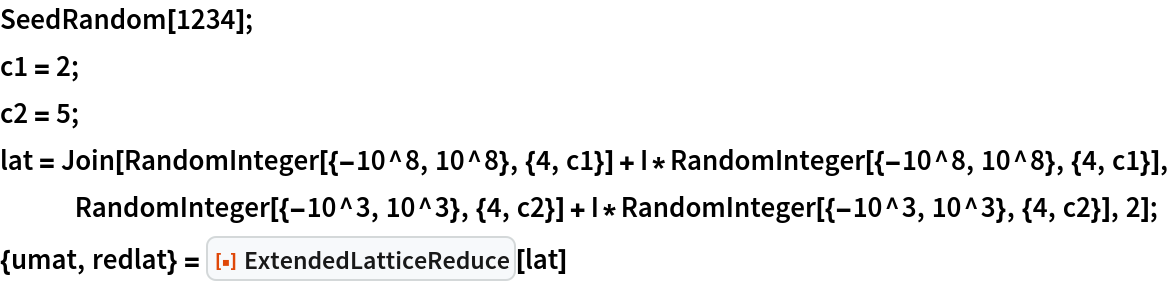Out=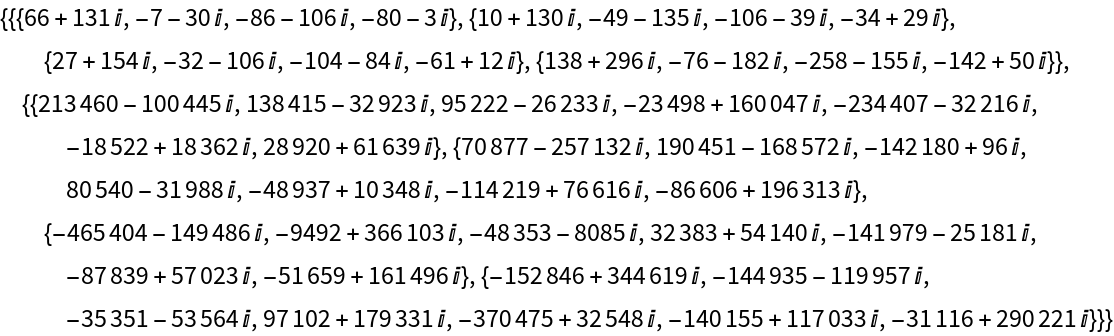Check the result:

 In:=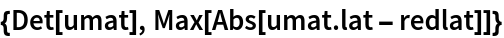Out=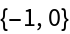### Options (7)

#### ReductionLambda (7)

Create a large matrix with big integers in the first two columns and an identity matrix on the right: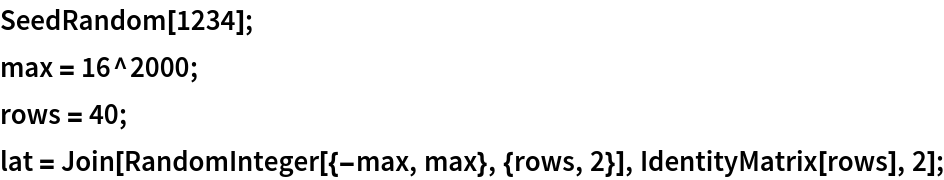First compute the default reduction:

 In:=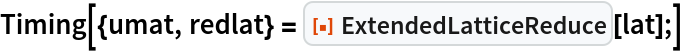Out=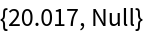Check the conversion matrix criteria:

 In:=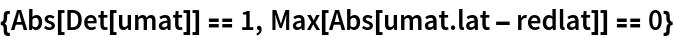Out=Computing a reduction of higher quality typically takes longer:

 In:=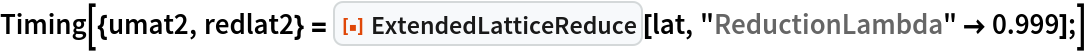Out=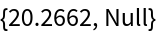Using a smaller reduction size ratio setting typically gives a faster computation:

 In:=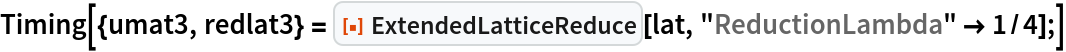Out=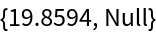The higher quality reduction manifests by most rows in the “stronger” result having norms smaller than for corresponding rows in the default reduction:

 In:=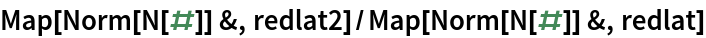Out=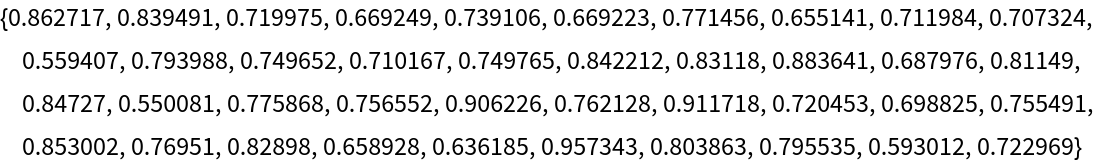The lower quality reduction manifests by most rows in the “weaker” result having norms larger than for corresponding rows in the default reduction:

 In:=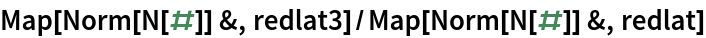Out=